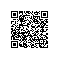# 关于JS中的constructor与prototype的总结

## 定义和用法

prototype 属性使您有能力向对象添加属性和方法

constructor 属性返回对创建此对象的数组函数的引用

### 语法

object.prototype.name=value

object.constructor

function Person(name){
console.log(name);
}
Person('js');   //js 

JavaScript内部会执行如下几个动作：

var f = new F();
alert(f.constructor === F.prototype.constructor);// output true 

if(f.constructor === F) {
// do sth with F
}  

if(f instanceof F) {
// do sth with F
} 

function Person(name){
this.name=name;
this.showMe=function(){
}
};
var one=new Person('<a href="#" class='replace_word' title="JavaScript知识库" target='_blank'>JavaScript</a>');
one.showMe();   //<a href="#" class='replace_word' title="JavaScript知识库" target='_blank'>javascript</a> 

function a(c){
this.b = c;
this.d =function(){
}
}
var obj = new a('test');
console.log(typeof obj.prototype);   //undefine
console.log(typeof a.prototype);   //object
console.log(a.prototype);
console.log(obj.__proto__ === a.prototype)   //true

a.prototype 包含了2个属性，一个是constructor ，另外一个是__proto__。这个constructor 就是我们的构造函数a，那么__proto__ 是什么呢？

var obj={};    也就是说，初始化一个对象obj。
obj.__proto__=a.prototype;
a.call(obj);   也就是说构造obj，也可以称之为初始化obj

function a(c){
this.b = c;
this.d =function(){
console.log(this.b);
}
}
a.prototype.test = function(){
console.log(this.b);
}
var obj = function (){}
obj.prototype = new a('test');
obj.prototype.test1 =function(){
console.log(22222);
}
var t = new obj('test');
t.test();   //console.log('test');

var t = new obj('test'); 我们可以得到 t.__proto__ = obj.prototype,但是上面指定obj.prototype =new a('test'); 可以这样来看下

obj.prototype = p, p = new a('test'); p.__proto__ = a.prototype;

function a(c){
this.b = c;
this.d =function(){
console.log(this.b);
}
}
var obj  = new a('test');
console.log(obj.constructor);//function a(){}
console.log(a.prototype.constructor);//function a(){}

a.prototype中寻找，而 a.prototype.constructor 是就a，所有两者的结果是一一样的

function Person(name){
this.name=name;
this.showMe=function(){
console.log(this.name);
}
};
Person.prototype.from=function(){
console.log('I come from prototype.');
}
function SubPerson(){}
SubPerson.prototype=new Person();
var subOne=new SubPerson();
subOne.from();//I come from prototype.
console.log(subOne.constructor);//function Person(name) {...};
console.log(SubPerson.prototype.constructor);//function Person(name) {...};  

function Person(name){
this.name=name;
this.showMe=function(){
console.log(this.name);
}
};
Person.prototype.from=function(){
console.log('I come from prototype.');
}
var father=new Person('js');//为了下面演示使用showMe方法，采用了js参数,实际多采用无参数
console.log(father.constructor);//查看构造函数,结果是:function Person(name) {...};
function SubPer(){}
SubPer.prototype=father;//注意这里
SubPer.prototype.constructor=SubPer;
var son=new SubPer();
son.showMe();//js
son.from();//I come from prototype.
console.log(father.constructor);//function SubPer(){...}
console.log(son.constructor);//function SubPer(){...}
console.log(SubPer.prototype.constructor);//function SubPer(){...}  

function F() {}
F.prototype = {
_name: 'Eric',
getName: function() {
return this._name;
}
};  

var f = new F();
console.log(f.constructor === F); // output false  

console.log(typeof f.constructor == 'undefined');// output false

alert(f.constructor === Object);// also output true

function F() {}
F.prototype = {
constructor: F, /* reset constructor */
_name: 'Eric',
getName: function() {
return this._name;
}
};  

var f = new F();
alert(f.constructor === F); // output true this time ^^  

alert(typeof Array.constructor != 'undefined');// output true

alert(typeof Array.constructor != 'undefined');// output true
alert(typeof Array.prototype.constructor === Array); // output true

alert(Array.constructor === Function);// output true
alert(Function.constructor === Function); // output true使用钉钉扫一扫加入圈子
+ 订阅